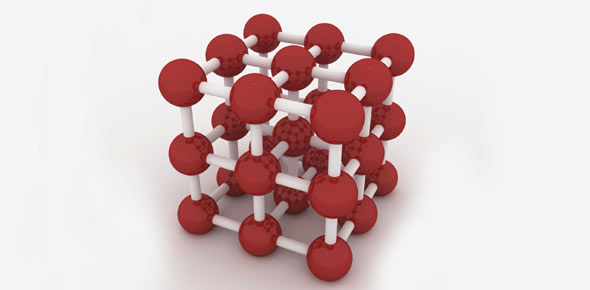# Names Of Common Polyatomic Ions

10 Questions | Total Attempts: 26Settings.

Related Topics
• 1.
How many molecules is 1 mole
• A.

1.67 x 10 ^-24

• B.

6.673 x 10^-11

• C.

9 x 10^9

• D.

6.02 x 10^23

• E.

3.0 x 10^8

• 2.
Which on of these is an oxyacid
• A.

Hydrochloric Acid

• B.

Acetic Acid

• C.

Hydrocyanic Acid

• D.

Hydrofluoric Acid

• E.

Hydroiodic Acid

• 3.
Ca(OH)2 is call Calcium (II) Hydroxide
• A.

True

• B.

False

• 4.
Name This Compound Mn(ClO4)7
• A.

Manganese (VII) Chlorite

• B.

Manganese (VII) Chlorate

• C.

Manganese (VII) hypochlorite

• D.

Manganese (VII) Hypochlorus

• E.

Manganese (VII) Perchloate

• 5.
• A.

Melting, Freezing

• B.

Melting Boiling

• C.

Evaporation Condensation

• D.

Sublimation Deposition

• E.

Deposition Esterification

• 6.
Perfume, Gasoline, Ethanol, Formaldehyde, Acetones  are example of
• A.

Aldehyes

• B.

Ketone

• C.

Alcohol

• D.

Volatile Liquids

• E.

Hydrocarbons

• 7.
• A.

Collisions are elastic

• B.

All Gases are nonpolar

• C.

Kinetic energy depends on Temperature

• D.

There are no attractive forces

• E.

Particles are always moving

• 8.
Tick the assumptions of the Kinetic theory
• A.

All Gases are Nonpolar

• B.

There are no attractive forces between molecules

• C.

Every container can contain 6.02 x 10^23 moles of gass

• D.

Kinetic Energy Depends on temperature

• E.

Polar Gases Move in Projectile motion

• F.

All Collisions are Elastic

• G.

Particles are all moving randomly.

• H.

Gases can move in circular motion

• I.

Gases consist of many tiny particles

• J.

No Gas can make hydrogen bonds

• K.

Vx of Gases are constant